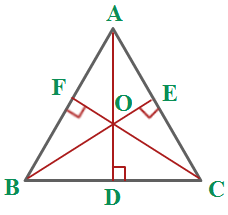# Orthocenter Formula

The orthocenter is the intersecting point for all the altitudes of the triangle. The point where the altitudes of a triangle meet is known as the Orthocenter. In the below mentioned diagram orthocenter is denoted by the letter ‘O’. There is no direct formula to calculate the orthocenter of the triangle. It lies inside for an acute and outside for an obtuse triangle.

Altitudes are nothing but the perpendicular line ( AD, BE and CF ) from one side of the triangle ( either AB or BC or CA ) to the opposite vertex. Vertex is a point where two line segments meet ( A, B and C ).To Calculate the slope of the sides of the triangle. The formula to calculate the slope is given as,

$\large Slope\;of\;a\;Line=\frac{y_{2}-y_{1}}{x_{2}-x_{1}}$

To calculate the perpendicular slope of the sides of the triangle. It gives us the slope of the altitudes of the triangle. The formula to calculate the perpendicular slope is given as,

$\large Perpendicular\;Slope\;of\;a\;Line=\frac{-1}{Slope\;of\;a\;Line}$

To calculate the equation for the altitudes with their respective coordinates. The point-slope formula is given as,

$\large y-y_{1}=m(x-x_{1})$

Finally, by solving any two altitude equations, we can get the orthocenter of the triangle.

### Solved Example

Question: Find the orthocenter of a triangle when their vertices are A(1, 2), B(2, 6), C(3, -4).

Solution:

Given, the vertices of the triangle,

A = (1, 2)
B = (2, 6)
C = (3, -4)

Slope of AB

=

$$\begin{array}{l}\frac{y_{2}-y_{1}}{x_{2}-x_{1}}\end{array}$$

=

$$\begin{array}{l}\frac{6 − 2}{2 – 1}\end{array}$$

=

$$\begin{array}{l}\frac{4}{1}\end{array}$$
= 4

Slope of CF

= Perpendicular slope of AB

=

$$\begin{array}{l}\frac{-1}{Slope of AB}\end{array}$$

=

$$\begin{array}{l}\frac{-1}{4}\end{array}$$

The equation of CF is given as,

y – y1 = m(x – x1)

y + 4 =

$$\begin{array}{l}\frac{-1}{4}\end{array}$$
(x – 3)

4y + 16 = -x + 3

x + 4y = -13 ——————————– (1)

Slope of BC

$$\begin{array}{l}\frac{y_{2} – y_{1}}{x_{2} – x_{1}}\end{array}$$

=

$$\begin{array}{l}\frac{-4 – 6}{3 – 2}\end{array}$$

=

$$\begin{array}{l}\frac{-10}{1}\end{array}$$
= -10

Perpendicular slope of BC

=

$$\begin{array}{l}\frac{-1}{Slope of BC}\end{array}$$

=

$$\begin{array}{l}\frac{-1}{-10}\end{array}$$

=

$$\begin{array}{l}\frac{1}{10}\end{array}$$

The equation of AD is given as,

y – y1 = m(x – x1)

y – 2 = 1/10(x – 1)

10y – 20 = x – 1

x – 10y = -19 ——————————– (2)

Subtracting equation (2) and (1),

x  + 4y  = -13

-x  + 10y =  19

——————

14y = 6

y = 6/14 = 3/7

Or

y = 0.429

Substituting the value of y in equation (1),

x + 4y = -13

x + 4(0.429) = -13

x + 1.716 = -13

x = -14.716

Orthocenter = (-14.716, 0.429)